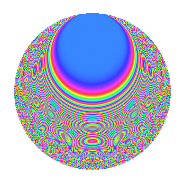# Properties

 Label 731.2.j.aLevel 731 Weight 2 Character orbit 731.j Analytic conductor 5.837 Analytic rank 0 Dimension 128 CM No

# Related objects

## Newspace parameters

 Level: $$N$$ = $$731 = 17 \cdot 43$$ Weight: $$k$$ = $$2$$ Character orbit: $$[\chi]$$ = 731.j (of order $$6$$ and degree $$2$$)

## Newform invariants

 Self dual: No Analytic conductor: $$5.83706438776$$ Analytic rank: $$0$$ Dimension: $$128$$ Relative dimension: $$64$$ over $$\Q(\zeta_{6})$$ Sato-Tate group: $\mathrm{SU}(2)[C_{6}]$

## $q$-expansion

The dimension is sufficiently large that we do not compute an algebraic $$q$$-expansion, but we have computed the trace expansion.

 $$\operatorname{Tr}(f)(q) =$$ $$128q - 4q^{2} + 116q^{4} - 12q^{8} + 70q^{9} + O(q^{10})$$ $$\operatorname{Tr}(f)(q) =$$ $$128q - 4q^{2} + 116q^{4} - 12q^{8} + 70q^{9} + 4q^{13} - 12q^{15} + 76q^{16} + 2q^{17} - 16q^{18} - 2q^{19} - 20q^{21} + 60q^{25} - 2q^{26} - 28q^{30} - 48q^{32} + 22q^{33} - 18q^{34} - 112q^{35} + 36q^{36} - 40q^{38} + 36q^{42} + 10q^{43} + 36q^{47} + 52q^{49} + 16q^{50} + 10q^{51} + 10q^{52} + 24q^{55} - 12q^{59} - 78q^{60} + 36q^{64} + 14q^{66} + 10q^{67} - q^{68} - 64q^{70} - 68q^{72} - 22q^{76} - 28q^{77} - 20q^{81} - 6q^{83} + 32q^{84} + 6q^{85} - 58q^{86} + 32q^{87} + 36q^{89} + 6q^{93} + 132q^{94} - 48q^{98} + O(q^{100})$$

## Embeddings

For each embedding $$\iota_m$$ of the coefficient field, the values $$\iota_m(a_n)$$ are shown below.

For more information on an embedded modular form you can click on its label.

Label $$a_{2}$$ $$a_{3}$$ $$a_{4}$$ $$a_{5}$$ $$a_{6}$$ $$a_{7}$$ $$a_{8}$$ $$a_{9}$$ $$a_{10}$$
135.1 −2.78045 −1.68248 0.971380i 5.73090 1.18797 + 0.685872i 4.67805 + 2.70087i −2.90625 + 1.67793i −10.3736 0.387158 + 0.670578i −3.30308 1.90703i
135.2 −2.78045 1.68248 + 0.971380i 5.73090 −1.18797 0.685872i −4.67805 2.70087i 2.90625 1.67793i −10.3736 0.387158 + 0.670578i 3.30308 + 1.90703i
135.3 −2.53253 −1.51123 0.872510i 4.41371 −3.60596 2.08190i 3.82724 + 2.20966i 0.435566 0.251474i −6.11280 0.0225487 + 0.0390554i 9.13221 + 5.27248i
135.4 −2.53253 1.51123 + 0.872510i 4.41371 3.60596 + 2.08190i −3.82724 2.20966i −0.435566 + 0.251474i −6.11280 0.0225487 + 0.0390554i −9.13221 5.27248i
135.5 −2.51145 −1.76122 1.01684i 4.30737 1.18626 + 0.684888i 4.42321 + 2.55374i 3.84346 2.21902i −5.79485 0.567928 + 0.983681i −2.97923 1.72006i
135.6 −2.51145 1.76122 + 1.01684i 4.30737 −1.18626 0.684888i −4.42321 2.55374i −3.84346 + 2.21902i −5.79485 0.567928 + 0.983681i 2.97923 + 1.72006i
135.7 −2.11821 −2.72590 1.57380i 2.48683 −0.994359 0.574094i 5.77403 + 3.33364i −0.0492215 + 0.0284181i −1.03120 3.45368 + 5.98195i 2.10626 + 1.21605i
135.8 −2.11821 2.72590 + 1.57380i 2.48683 0.994359 + 0.574094i −5.77403 3.33364i 0.0492215 0.0284181i −1.03120 3.45368 + 5.98195i −2.10626 1.21605i
135.9 −2.09187 −0.414315 0.239205i 2.37591 1.22310 + 0.706158i 0.866692 + 0.500385i −2.02420 + 1.16867i −0.786343 −1.38556 2.39986i −2.55857 1.47719i
135.10 −2.09187 0.414315 + 0.239205i 2.37591 −1.22310 0.706158i −0.866692 0.500385i 2.02420 1.16867i −0.786343 −1.38556 2.39986i 2.55857 + 1.47719i
135.11 −2.05109 −2.62751 1.51699i 2.20698 2.73044 + 1.57642i 5.38926 + 3.11149i 0.592018 0.341802i −0.424527 3.10253 + 5.37374i −5.60038 3.23338i
135.12 −2.05109 2.62751 + 1.51699i 2.20698 −2.73044 1.57642i −5.38926 3.11149i −0.592018 + 0.341802i −0.424527 3.10253 + 5.37374i 5.60038 + 3.23338i
135.13 −2.04963 −1.14468 0.660879i 2.20099 −1.07261 0.619273i 2.34616 + 1.35456i 0.325723 0.188056i −0.411958 −0.626478 1.08509i 2.19846 + 1.26928i
135.14 −2.04963 1.14468 + 0.660879i 2.20099 1.07261 + 0.619273i −2.34616 1.35456i −0.325723 + 0.188056i −0.411958 −0.626478 1.08509i −2.19846 1.26928i
135.15 −1.73933 −1.10646 0.638817i 1.02526 2.28826 + 1.32113i 1.92450 + 1.11111i −4.26158 + 2.46042i 1.69540 −0.683825 1.18442i −3.98003 2.29787i
135.16 −1.73933 1.10646 + 0.638817i 1.02526 −2.28826 1.32113i −1.92450 1.11111i 4.26158 2.46042i 1.69540 −0.683825 1.18442i 3.98003 + 2.29787i
135.17 −1.28957 −0.316022 0.182455i −0.337012 3.24573 + 1.87392i 0.407532 + 0.235289i 2.23124 1.28820i 3.01374 −1.43342 2.48276i −4.18560 2.41656i
135.18 −1.28957 0.316022 + 0.182455i −0.337012 −3.24573 1.87392i −0.407532 0.235289i −2.23124 + 1.28820i 3.01374 −1.43342 2.48276i 4.18560 + 2.41656i
135.19 −1.28732 −1.78909 1.03293i −0.342809 0.933959 + 0.539221i 2.30313 + 1.32971i 3.12985 1.80702i 3.01594 0.633889 + 1.09793i −1.20230 0.694150i
135.20 −1.28732 1.78909 + 1.03293i −0.342809 −0.933959 0.539221i −2.30313 1.32971i −3.12985 + 1.80702i 3.01594 0.633889 + 1.09793i 1.20230 + 0.694150i
See next 80 embeddings (of 128 total)
 $$n$$: e.g. 2-40 or 990-1000 Embeddings: e.g. 1-3 or 509.64 Significant digits: Format: Complex embeddings Normalized embeddings Satake parameters Satake angles

## Inner twists

This newform does not have CM; other inner twists have not been computed.

## Hecke kernels

There are no other newforms in $$S_{2}^{\mathrm{new}}(731, [\chi])$$.